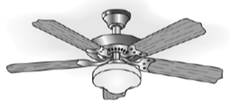Chapter 6.1, Problem 27EElementary Geometry For College St...

7th Edition
Alexander + 2 others
ISBN: 9781337614085

Solutions

Chapter
SectionElementary Geometry For College St...

7th Edition
Alexander + 2 others
ISBN: 9781337614085
Textbook Problem

A ceiling fan has equally spaced blades. What is the measure of the angle formed by two consecutive blades if there are a) 5 blades? b) 6 blades?To determine

(a)

To Find: The measure of the angle formed by two consecutive blades if there are 5 blades.

Explanation

Given:

A ceiling are equally spaced 5 blades.

Postulate used:

Central angle postulate:

In a circle, the degree measure of a central angle is equal to the degree measure of its intercepted arc.

Congruent arcs:

In a circle, congruent arcs are arcs with equal measures.

Since, the sum of the measures of the consecutive arc that form a circle is 360o.

Calculation:

Consider, the figure given below:

Since, the 5 blades are equally spaced.

Therefore, in a circle, congruent arcs are arcs with equal measures.

mAB=mBC

mBC=mCD

mCD=mDE

And

mDE=mEA

Since, the sum of the measures of the consecutive arc that form a circle is 360o.

mAB+mBC+mCD+mDE+mEA=360o

By using the above result we obtain

To determine

(b)

To calculate:

The measure of the angle formed by two consecutive blades if there are 6 blades.

Still sussing out bartleby?

Check out a sample textbook solution.

See a sample solution

The Solution to Your Study Problems

Bartleby provides explanations to thousands of textbook problems written by our experts, many with advanced degrees!

Get Started

Simplify: 1236

Elementary Technical Mathematics

Evaluate the expression sin Exercises 116. 323

Finite Mathematics and Applied Calculus (MindTap Course List)

By definition the improper integral It is not an improper integral.

Study Guide for Stewart's Single Variable Calculus: Early Transcendentals, 8th

True or False: These lines are skew:

Study Guide for Stewart's Multivariable Calculus, 8th